# Triangles Class 10 Maths notes

In this page we will explain the topics for the chapter 6 of Triangles Class 10 Maths.We have given quality Arithmetic Progressions Class 10 Notes to explain various things so that students can benefits from it and learn maths in a fun and easy manner, Hope you like them and do not forget to like , social share and comment at the end of the page.
Table of Content

## Similar Figures

Two figures having the same shape but not necessarily the same size are called similar figures.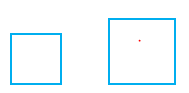Examples:
All circles are similar
All square are similar
All equilateral triangles are similar
All the congruent figures are similar but the converse is not true

## Similar Polygons

Two polygons with same number of sides are said to be similar, if
(i) their corresponding angles are equal and
(ii) their corresponding sides are in proportion (or are in the same ratio).

## Basic Proportionally Theorem (or Thales Theorem)

If a line is drawn parallel to one side of a triangle intersecting the other two sides, then it divides the two sides in the same ratio.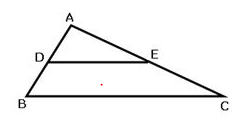In the $\Delta ABC \; , \;if \; DE \; || \; BC$
i) $\frac {AD}{DB}= \frac {AE}{EC}$
ii) $\frac {AD}{AB}= \frac {AE}{AC}$
iii) $\frac {DB}{AB}= \frac {EC}{AC}$
Proof:
Construction: Join BE and CD. Draw DM ⊥ AC and EN ⊥ AB
Consider $\Delta ADE \; and \; \Delta BDE$
$ar(\Delta ADE) =\frac {1}{2} \times AD \times EN$
$ar(\Delta BDE) =\frac {1}{2} \times DB \times EN$

$\frac {ar( \Delta ADE) }{ar( \Delta BDE)} =\frac {(1/ 2 \times AD \times DM )}{(1/ 2 \times DB \times DM)} =\frac {AD}{ DB}$ ...........(1)

Consider $\Delta ADE \; and \; \Delta DEC$

$ar(\Delta ADE) =\frac {1}{2} \times AE \times DM$
$ar(\Delta DEC) =\frac {1}{2} \times EC \times DM$

$\frac {ar( \Delta ADE) }{ar( \Delta DEC)} =\frac { 1/ 2 \times AE \times DM)}{ (1/ 2 \times EC \times DM)} = \frac {AE}{ EC}$ ..........(2)

Consider $\Delta BDE \; and \; \Delta CED$

$ar(\Delta BDE) = ar(\Delta CED)$ .........(3)

[Since $\Delta BDE \; and \; \Delta CED$ are on the same base, DE, and between the same parallels, BC and DE.]

Therefore
$\frac {AD}{DB}= \frac {AE}{EC}$ [From (1), (2) and (3)]
Converse of Basic Proportionality Theorem:
If a line divides any two sides of a triangle in the same ratio, then the line is parallel to the third side.

## Criteria for Similarity of Triangles

Now triangle is also type of polygon and we already know the similarity criteria for that. So Two triangles are said to be similar, if
(i) their corresponding angles are equal and
(ii) their corresponding sides are in proportion (or are in the same ratio).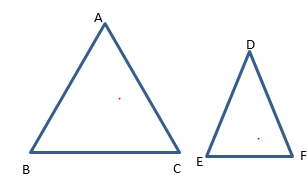Corresponding angles are equal
$\angle A=\angle D,\angle B= \angle E,\angle C=\angle F$
Corresponding sides are in proportion (or are in the same ratio).
$\frac {AB}{DE} = \frac {BC}{EF} = \frac {AC}{DF}$
Symbolically, we write the similarity of these two triangles as
$\Delta ABC \sim \Delta DEF$
The symbol ‘~’ stands for ‘is similar to’. Recall that you have used the symbol ‘≅’ for ‘is congruent to’ in Class IX
We should keep the letters in correct order on both sides

## Different Criterion for similarity of the triangles

(i) AA or AAA Similarity Criterion: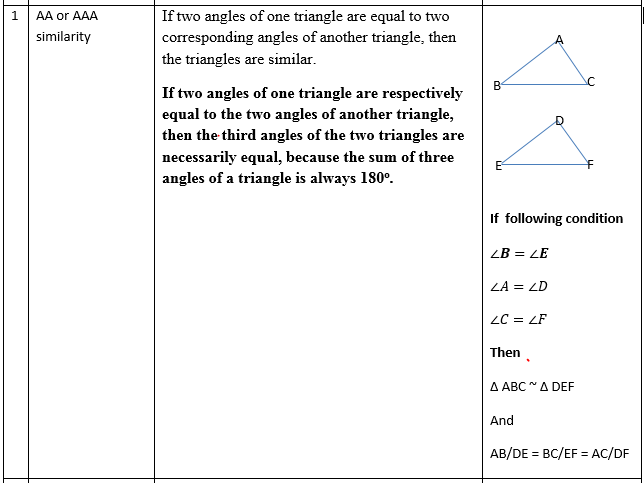(ii) SSS Similar Criterion: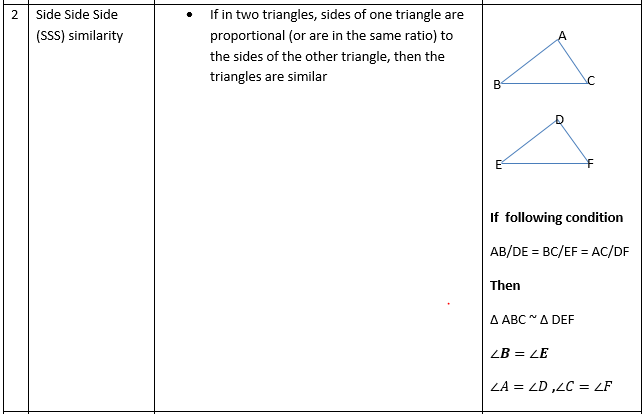We may recall that the below two conditions
(i) corresponding angles are equal and
(ii) corresponding sides are in the same ratio
are required  for two polygons to be similar
However, on the basis of last two SSS and AAA criterion you can now say that in case of similarity of the two triangles, it is not necessary to check both the conditions as one condition implies the other.
(iii) SAS Similar Criterion: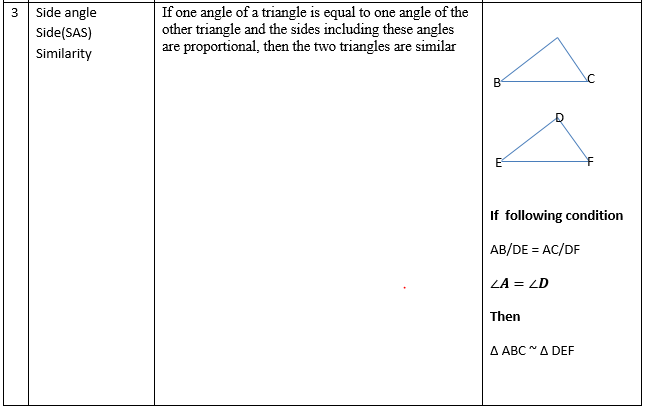## Solved Examples

1) if PQ || RS, prove that $\Delta POQ \sim \Delta SOR$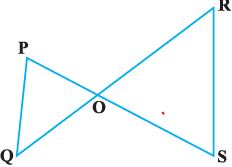Solution : Given PQ || RS
So, $\angle P = \angle S$ (As per Alternate angles)
and $\angle Q = \angle R$
Also, $\angle POQ = \angle SOR$ (As they are Vertically opposite angles)
Therefore, $\Delta POQ \sim \Delta SOR$ (AAA similarity criterion)
2) The side lengths of  $\Delta PQR$ are 16, 8, and 18, and the side lengths of $\Delta XYZ$ are 9, 8, and 4. Find out if the triangle is similar
Solution
For these questions , it is recommended to compare the ratio of largest side and shortest side and then remaining side
So 18/9=2 ( Longest side)
8/4=2 ( Shortest side)
16/8=2 ( Remaining side)
Since all ratio's are equal , triangles  are similar
3) In $\Delta ABC,\; \angle A = 22^0 \; and \; \angle B = 68^0$. In $\Delta DEF, \angle D = 22^0 \; and \; \angle F = 90^0$
Solution:
For $\Delta ABC$,three angles are
$\angle A = 22^0$ and $\angle B = 68^0$
$\angle C= 180 - ( \angle A+ \angle B) = 90$
For $\Delta DEF$,three angles are
$\angle D = 22^0 \; and \; \angle F = 90^0$
$\angle E= 180 - ( \angle D+ \angle F) = 68$
So from AAA similarity criterion, triangles are similar
$\Delta ABC \sim \Delta DEF$

## Areas of Similar Triangles

The ratio of the areas of two similar triangles is equal to the square of the ratio of their corresponding sides.
.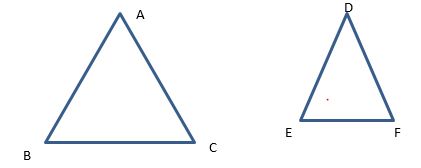Given $\Delta ABC \sim \Delta DEF$
$\frac {ar (ABC)}{ar (DEF)} =\frac {AB^2}{DE^2} = \frac {BC^2}{EF^2} = \frac {AC^2}{DF^2}$
Also
The ratio of the areas of two similar triangles is equal to the ratio of the squares of their corresponding altitudes.
The ratio of the areas of two similar triangles is equal to the sum of the squares of their corresponding angle bisectors.
Example
1) Let $\Delta ABC ~\sim \Delta DEF$ and their areas be, respectively, 64 cm2 and 121 cm2. If EF = 15.4 cm, find BC.
Solution:
We know that
$\frac {ar( ABC)}{ar( DEF)}= \frac {BC^2}{EF^2}$
$\frac {64}{121}=\frac {BC^2}{15.4^2}$
BC= 11.2 cm

## Pythagoras Theorem:

In a right triangle, the square of the hypotenuse is equal to the sum of the squares of the other two sides.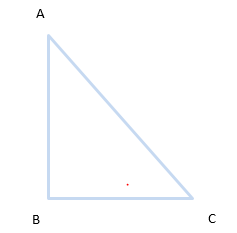$AC^2 = AB^2 +BC^2$

How to prove it using paper and pencil
1. Take a card board of size say 20 cm x 20 cm.
2. Cut any right angled triangle and paste it on the cardboard. Suppose that its sides are a, b and c.
3. Cut a square of side a cm and place it along the side of length a cm of the right angled triangle.
4. Similarly cut squares of sides b cm and c cm and place them along the respective sides of the right angles triangle.
5. Color the diagram as shown in below figure. You can choose any other color if you want
6. Join BH and AI. These are two diagonals of the square ABIH. The two diagonals intersect each other at the point O.
7. Through O, draw $RS || BC$.
8. Draw PQ, the perpendicular bisector of RS, passing through O.
9. Now the square ABIH is divided in four quadrilaterals. Colour them as shown in figure.
10. From the square ABIH cut the four quadrilaterals.
11. Now arrange them as given above and You will see the sum of square of a and b matches square of c in second figure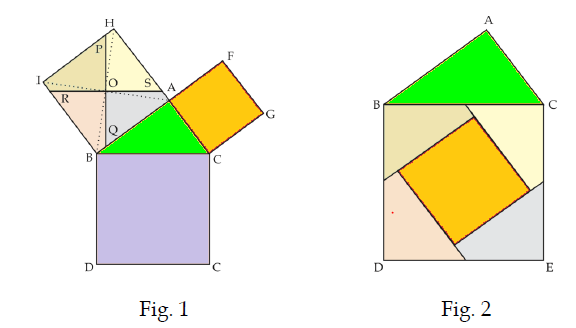Practice Questions
Verify if these are sides of Right angle triangle
• 3,4,5
• 5,12,13
• 7,24,25
• 20,21,29
• 12,16,20
• 15,36,39
• 10,24,26
• 4,5,8

## Converse of Pythagoras Theorem:

In a triangle, if square of one side is equal to the sum of the squares of the other two sides, then the angle opposite the first side is a right angle.
If a perpendicular is drawn from the vertex of the right angle of a right triangle to the hypotenuse, the triangles on each side of the perpendicular are similar to the whole triangle and similar to each other.
i. e., if in triangle ABC,
$\angle B = 90$ and BD = AC, then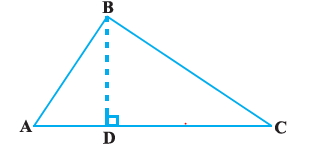(i) $\Delta ADB \sim \Delta ABC$
(ii) $\Delta BDC \sim \Delta ABC$
(iii) $\Delta ADB \sim \Delta BDC$

RHS Similarity Criterion:
If in two right triangles, hypotenuse and one side of one triangle are proportional to the hypotenuse and one side of the other triangle, then the two triangles are similar by RHS similarity criterion.

### Quiz Time

Question 1 Sides of two similar triangles are in the ratio 4:9.What is the ratio of areas of these triangles ?
A)4:9
B) 2: 3
C) 16: 81
D) None of the above
Question 2 In a right angle triangle shown below ?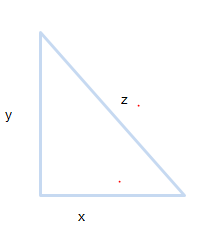A) $x^2 +y^2 =z^2$
B) $x^2 +z^2 =y^2$
C) $z^2 +y^2 =y^2$
D) $x +y =z$
Question 3 In triangle ABC and PQR , $\angle A= \angle R$ and $\angle B= \angle P$ then
A) $\Delta ABC \sim \Delta RQP$
B) $\Delta ABC \sim \Delta PRQ$
C) $\Delta ABC \sim \Delta PQR$
D) $\Delta ABC \sim \Delta RPQ$
Question 4 If in two triangles, the corresponding angles are equal, their corresponding sides.
A) equal
B) proportional
C) square of other side
D) none of these
Question 5 If a line divides two sides of a triangle in the same ratio, the line is?
A) parallel to third side
B) perpendicular to third side
C)equal to third side
D) none of the above
Question 6 Check the correct answer ?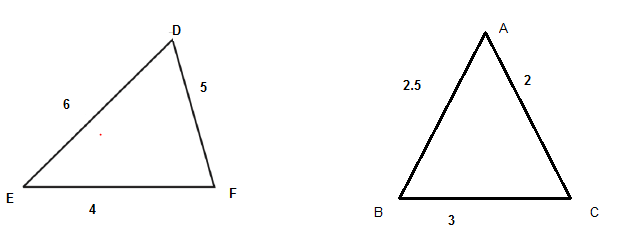A) $\Delta ABC \sim \Delta DFE$
B) $\Delta ABC \sim \Delta EFD$
C) $\Delta ABC \sim \Delta DEF$
D) $\Delta ABC \sim \Delta FDE$

## Summary

Here is Similar Triangles Class 10 Maths Notes Summary
• Two triangles are similar if the correesponding angles are equal and their corresponding sides are in the same ratio or proportion
• A line parallel to one edge of a triangle intersects the other two sides in distinct points, then other two sides are split in the same proportion.
• If a line divides any two sides of a triangle in the same ratio, then the line is parallel to the third side.
• If the corresponding angles are two triangles are same,then as AAA Similarity criterion, the triangles are similar and the corresponding sides are in the same proportion.
• If the corresponding sides are two triangles are in same proprtion,then as SSS Similarity criterion, the triangles are similar and the corresponding angles are equal
• If one angle of a triangle is equal to one angle of another triangle and the sides including these angles are in the same ratio (proportional), then the triangles are similar as per SAS similarity criterion
• All congurent triangles are similar but all similar triangles are not congruentGo back to Class 10 Main Page using below links

### Practice Question

Question 1 What is $1 - \sqrt {3}$ ?
A) Non terminating repeating
B) Non terminating non repeating
C) Terminating
D) None of the above
Question 2 The volume of the largest right circular cone that can be cut out from a cube of edge 4.2 cm is?
A) 19.4 cm3
B) 12 cm3
C) 78.6 cm3
D) 58.2 cm3
Question 3 The sum of the first three terms of an AP is 33. If the product of the first and the third term exceeds the second term by 29, the AP is ?
A) 2 ,21,11
B) 1,10,19
C) -1 ,8,17
D) 2 ,11,20